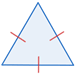# Scalene triangle

A scalene triangle is a triangle in which none of the three sides or angles are equal.

In the figure above, the differing number of tick marks and arcs indicate that each side and angle of the triangle has a different measure. This is commonly used notation in geometry; sides that are the same length (congruent) are indicated using the same number of tick marks, and angles that are the same are indicated using the same number of arcs.

## Properties of scalene triangles

There are some properties that all triangles share:

• The sum of all internal angles of a triangle is equal to 180°.
• The length of the side of a triangle corresponds to the size of the angle opposite the side; the longer the side, the larger the angle. It is worth noting that the ratios do not correspond: if side a is twice the length of b, it does not mean that angle A is twice the size of angle B.
• The sum of the length of any two sides of a triangle is greater than the length of the third side.

There are more properties that all triangles share, but these are a few commonly used properties in geometry.

Scalene triangles specifically have the following properties:

• No equal sides.
• No equal angles.
• No line of symmetry - a scalene triangle cannot be divided into two identical halves. In contrast, equilateral and isosceles triangles both have line(s) of symmetry.
• Scalene triangles can be right triangles, obtuse triangles, or acute triangles.

## Area and perimeter

There are a number of different ways to find the area of a triangle. The general formula for the area (A) of a triangle iswhere b is the base and h is the height of the triangle. This works for any triangle given that the information provided makes it possible to find the base and the height of the triangle.

For scalene triangles, since each side has a different length, in most cases the problem likely will provide the side lengths, or make it possible to find the side lengths. In cases where each side of a triangle is known, Heron's formula can be used to find the area of the triangle:In Heron's formula, a, b, and c represent the lengths of the sides of the triangle, and s is:Note that a, b, and c are labeled arbitrarily on the triangle. Any of the sides can be labeled a, b, or c. The triangle above can also be referenced for finding the perimeter of a triangle. To find the perimeter (P) of a triangle, simply find the sum of the length of each side of the triangle:

P = a + b + c

## Triangles categorized by their sides

Triangles are often categorized by either their sides or angles. A scalene triangle is one of three triangles classified by its sides.

TypeSidesFigure
Scalene no sides are equal in lengthIsosceles 2 sides are equal in lengthEquilateral all sides are equal in lengthTriangles are also often categorized as obtuse, acute, or right triangles based on their angles.